# Acceleration 2

if a car traveling at a velocity of 80 m/s/south accelerated to a velocity of 100 m/s east in 5 seconds, what is the cars acceleration? using Pythagorean theorem

Correct result:

a =  25.6125 m/s2

#### Solution:We would be pleased if you find an error in the word problem, spelling mistakes, or inaccuracies and send it to us. Thank you!Tips to related online calculators
Do you want to convert velocity (speed) units?
Do you want to convert time units like minutes to seconds?
Pythagorean theorem is the base for the right triangle calculator.

#### You need to know the following knowledge to solve this word math problem:

We encourage you to watch this tutorial video on this math problem:

## Next similar math problems:

• Up and down motionWe throw the body from a height h = 5 m above the Earth vertically upwards v0 = 10 m/s. How long before we have to let the second body fall freely from the same height to hit the Earth at the same time?
• Angled cyclist turnThe cyclist passes through a curve with a radius of 20 m at 25 km/h. How much angle does it have to bend from the vertical inward to the turn?
• Acceleration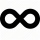Describe how the cyclist's acceleration changes on individual sections (sections AB plane, BC turn, CD plane, DA turn), which describes the trajectory in the shape of an eight at a constant speed. The speed on the cyclist's tachometer is constant
• Accelerated motion - mechanicsThe delivery truck with a total weight of 3.6 t accelerates from 76km/h to 130km/h in the 0.286 km long way. How much was the force needed to achieve this acceleration?
• GravitationFrom the top of the 80m high tower, the body is thrown horizontally with an initial speed of 15 m/s. At what time and at what distance from the foot of the tower does the body hit the horizontal surface of the Earth? (use g = 10 ms-2)
• Rocket start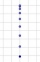The body launched vertically up returns to the start site in 6 seconds. What height did it have?
• Orlík hydroelectric plantThe Orlík hydroelectric power plant, built in 1954-1961, consists of four Kaplan turbines. For each of them, the water with a flow rate of Q = 150 m3/s is supplied with a flow rate of h = 70.5 m at full power. a) What is the total installed power of the p
• Free fallThe free fall body has gone 10m in the last 0.5s. Find the body speed at the moment of impact.
• Car overtaking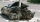A passenger car travels at a speed of 30 m/s, and before it travels freight truck that drives at a constant speed of 10 m/s. Within 30 meters of the truck, the driver of the car finds out that the truck can not overtake. That's why it starts braking with
• An accelerationThe car goes on a straight road at a speed of 72 km/h. At some point, the driver starts to brake and stops the car in 5 seconds. Find: (a) the acceleration during braking (b) the distance traveled during braking.
• CollisionThe two bodies, whose initial distance is 240 m, move evenly against each other consistently. The first body has an initial velocity of 4 m/s and an acceleration of 3 m/s2, the second body has an initial speed of 6 m/s and an acceleration of 2 m/s2. Fin
• Car crashOn the road, with a maximum permitted speed of 60 km/h, there was a car crash. From the length of the vehicle's braking distance, which was 40 m, the police investigated whether the driver did not exceed that speed. What is the conclusion of the police, a
• Free fall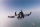How long does the stone fall freely into a depth of 80m? What speed will it hit the bottom of the abyss?
• Traffic collisionWhen investigating a traffic accident, it was found that the driver stopped the vehicle immediately after the accident by constant braking on a 150 m track in 15 seconds. Do you approve that the driver exceeded the permitted speed (50 km/h) in the village
• The tram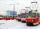The tram is moving with acceleration a = 0.3m/s2. How long it will pass the first meter of track? How long does it take 10 meters. What is its speed at the end of the 10 meters track?
• Forces on earth directionsA force of 60 N [North] and 80 N [East] is exerted on an object wigth 10 kg. What is the acceleration of the object?
• Hydrostatic forceWhat hydrostatic force is applied to an area of 30 cm2 in water at a depth of 20 m? (Water density is 1000 kg/m3)